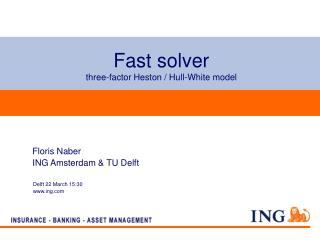DownloadDownload PresentationFast solver three-factor Heston / Hull-White model

# Fast solver three-factor Heston / Hull-White model

Télécharger la présentation## Fast solver three-factor Heston / Hull-White model

- - - - - - - - - - - - - - - - - - - - - - - - - - - E N D - - - - - - - - - - - - - - - - - - - - - - - - - - -
##### Presentation Transcript

1. Fast solver three-factor Heston / Hull-White model Floris Naber ING Amsterdam & TU Delft Delft 22 March 15:30 www.ing.com

2. Outline • Introduction to the problem (three-factor model) • Equity underlying • Stochastic interest • Stochastic volatility • Solving partial differential equations without boundary conditions • 1-dimensional Black-Scholes equation • 1-dimensional Hull-White equation • Conclusion • Future goals ING

3. Introduction (Three-factor model) • Underlying equity: S: underlying equity, r: interest rate, q:dividend yield, v:variance • Stochastic interest (Hull-White) r: interest rate, θ:average direction in which r moves, a:mean reversion rate,:annual standard deviation of short rate • Stochastic volatility (Heston) v:variance, λ:speed of reversion, :long term mean, η:vol. of vol. ING

4. Introduction Simulation Heston processSimulation Hull-White process (λ:1, :0.35^2, η:0.5,v0:0.35^2,T:1) (θ:0.07,a:0.05, σ:0.01, r0:0.03) ING

5. Introduction Pricing equationfor the three-factor Heston / Hull-White model: FAST ACCURATE GENERAL ING

6. Solving pde without boundary conditions Solving: • Implicitly with pde-boundary conditions: • whole equation as boundary condition using one-sided differences • Explicitly on a tree-structured grid ING

7. 1-dimensional Black-Scholes equation Black-Scholes equation: r: interest q: dividend yield σ: volatility V: option price S: underlying equity ING

8. Black-Scholes(solved implicitly with pde) • Inflow at right boundary, but one-sided differences wrong direction • Non-legitimate discretization, due to pde-boundary conditions (positive and negative eigenvalues) • Actually adjusting extra diffusion and dispersion at boundary ING

9. Black-Scholes (solved explicitly on tree) • Upwind is used, so accuracy might be bad • Strict restriction for stability of Euler forward • Upperbound for spacestep with Gerschgorin Example: r = 0.03, σ = 0.25, q = 0, S = [0,1000] gives N < 7 • Better time discretization methods needed, proposed RKC-methods. ING

10. 1-dimensional Hull-White equation Hull-White equation: r: interest rate θ:average direction in which r moves a:mean reversion rate :annual standard deviation of short rate ING

11. Hull-White (solved implicitly with pde) Caplets: ING

12. Hull-White (solved implicitly with pde) • Flow direction same as one-sided differences as long as • Discretization is not legitimate, but effects are hardly noticeable ING

13. Hull-White (solved explicitly on tree) • Transformation applied to get rid of ‘-rV’ • Upwind is used • Restriction on the time- and spacestep, but easier satisfied than Black-Scholes restriction • Results look accurate ING

14. Conclusion • Implicit methods with pde-boundary conditions: • Give problems due to: non legitimate discretization and wrong flow-direction • Put boundary far away to obtain accurate results • Explicit methods: • Very hard to satisfy stability conditions • Due to upwind less accurate ING

15. Future goals • More research on two methods to solve pdes • Explicit with RKC-methods • Investigating the Heston model • Implementing three-factor model solver ING